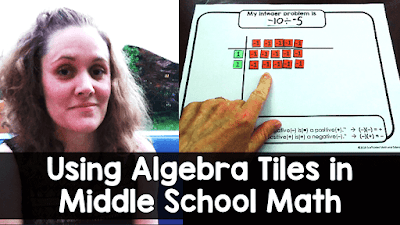## Pages

### A hands-on prime vs. composite numbers investigationIf your students struggle with the idea of prime vs. composite numbers, this hands-on investigation activity into prime numbers may be helpful, especially to the kinesthetic learners in your classroom.

The setup is easy: students cut out 100 squares to use as their algebra tiles. You can get the free 100 squares template here.

After cutting out their squares, students investigate different numbers to see if they make rectangles.

Since a prime number is cool in that the only numbers that divide into it are 1 and itself, if a number is prime we won't be able to make a rectangle from its number of squares manipulatives.

Let's start with 24. I counted our 24 squares to use in this investigation. At first it may seem that we can't make a rectangle... but we have to try more than one configuration.

And since we can make a rectangle from 24 squares, 24 is composite.

There only needs to be one way to make a rectangle to prove that a number is composite, but some numbers will of course make more than one rectangle. Some students may find 24's 2x12 configuration. If there's even one configuration that makes a rectangle, we have a composite number!

Is 23 prime? This configuration doesn't make a rectangle.

Neither does this one.

After a bunch of tries, we find that it is impossible to make a rectangle from 23 squares, so 23 must be prime.

The more students working on each number the better. This helps make sure no configurations are left out so that students can be sure a number is prime.

Let's try one more... 27. This isn't a rectangle.

Neither is this, but we're almost there....

24 squares makes a rectangle so 27 is composite.

Additional resources:

If you'd like to learn more about ways to use algebra tiles, I have put together an algebra tiles tutorial video that covers ways to use algebra tiles in middle school math.

### Using Algebra Tiles in Middle School Math:watch video

#### 12 comments:

1.How do you address 2 and 3? They are both prime and can make rectangles. Would we need to specify that if the only way to make a rectangle is one long line, then that number is prime?

1.Great question. Yes, a side of 1 can't count because it would show a factor of 1.

2.Great activity, thank you.

1.3.Love this activity! Thank you for sharing

1.My pleasure! I hope you have a wonderful winter break!

4.I love this activity for prime numbers and use it all the time with decks of cards.

1.That's neat that you use cards! I hadn't thought to do that.

5.How does this transfer to other higher numbers? My students do not know their multiplication/division factors. They are in Resource Room for math in 6th grade.

6.Good activity

7.Can we use for this for greater numbers more than 100

1.Definitely! I just kept it under 100 so the pieces would be manageable for my daughter to count. You could use any number of pieces.